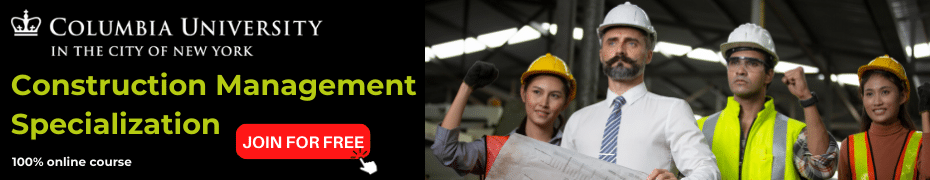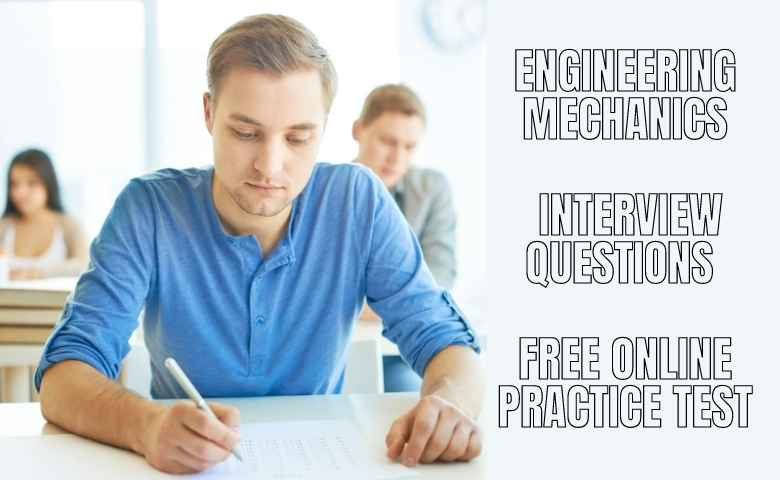ConstructionPlacementsPractice Tests

# Engineering Mechanics Interview Questions Free Online Practice Test

Last Updated on January 8, 2022 by Admin

Practice the below online practice test for the Engineering Mechanics Subject and determine how much you score before appearing for your following interview and written test. Kindly check Engineering Mechanics Interview Questions and take this Free Online Practice Test.

Take this Construction Manager job-oriented online test, get your score, learn about the basics and advanced concepts of Engineering Mechanics and improve your scores by practicing our engineering mechanics interview questions and answers. Take this engineering mechanics interview questions mock test.

If you score less than 7 in this Engineering Mechanics Free Online Practice Test, consider improving your essential competency skill in the engineering mechanics subject by considering these Civil Engineering online Courses

Take this online Engineering Mechanics interview questions practice test for Civil Engineer Job in 2022 and beyond.

## Welcome to your Engineering Mechanics Test

### Instructions:

• This is a FREE online test
• Total number of questions: 15
• The time allowed: 15 minutes
• Each question carry 1 mark, no negative marks
• DO NOT refresh the page
• All the best!
Forces passing through a common point are known as _____

Varignon's theorem is used to find ________

Couple is formed due to two ______

If a material has no uniform density throughout the body, then the position of centroid and center of mass are ________

During unidirectional motion, the displacement and distance traveled by a particle with uniform acceleration is ______

According to which law, every action has an equal and opposite reaction?

A man of 60 kg moves in a lift of constant velocity 5 m/s. What is the reactive force acting on the man by the elevator?

In projectile motion, which of the following factors affecting the actual path of motion are neglected?

What is the SI unit of linear momentum?

Which of the following conditions should be satisfied for co-planer concurrent forces to be in equilibrium?

According to Lami's theorem, a body is in equilibrium condition if each force among three ____________ are proportional to sine angle between other two.

Which motion has magnitude of static frictional force directly proportional to normal reaction?

Frictional force depends on ________

The force for which work done is independent of _______ is called as conservative force.

According to work energy principle, a particle of mass m when subjected to unbalanced force system, the work done during displacement by all forces is equal to change in ____________ during displacement.